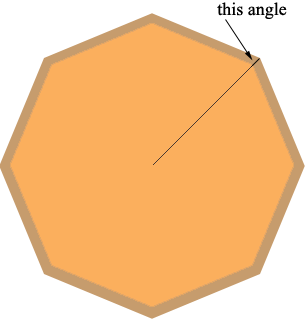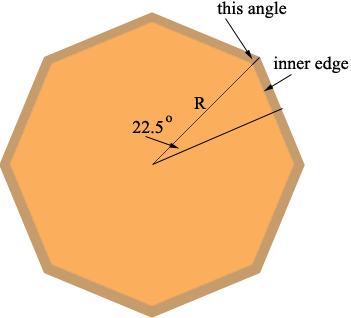SEARCH HOMEMath Central Quandaries & QueriesI have a circle photo 18 and one quarter inches round. I want to cut frame for it in a octagon shape. What would the angle and the length of cut be? MikeMike,

You will have to cut edges so that the cut meets one edge at an angle of 67.5 degreesAs for length, if it is 18.25" round it will be 5.8" across (similar to a cereal bowl) - is this what you mean? The radius R would be 2.9" and the inner edge would be given by 2R tan(22.5 degrees) which is 0.828 R or 2.4 inches (that's about 2 and 13/32 inches if you work in binary inches like most North American carpenters.)But this frame will look cramped, and there will be gaps near the corners. I would urge you to "mat" the photograph in a card mat - circular on the inside, octagonal on the outside - at least 2 inches wide. A 2" increase in radius would give a center-to-corner measurement of 4.9" and an inner edge of about 4 and 1/16 inches.

If I have misunderstood your initial measurements, or you want a different size of mat, you can follow these calculations to get the size you need.

Good Hunting!
RDMath Central is supported by the University of Regina and The Pacific Institute for the Mathematical Sciences.